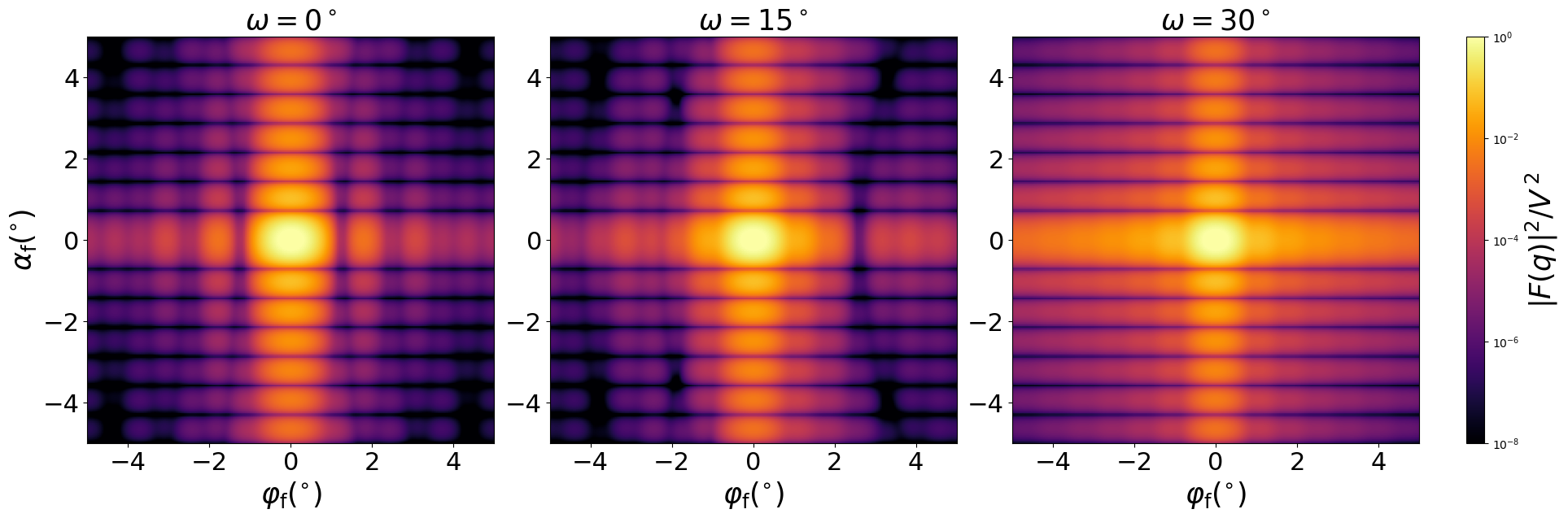### Prism3

A prism based on equlateral triangle.#### Constructor

Prism3(L, H)


Parameters:

• L, edge length of the trigonal base
• H, height

#### Usage

As for any other Form factor.

#### Implementation

Class Prism3 inherits from the interface class IFormFactor .

Form factor is computed as

$$F(\mathbf{q})=H \space \exp\Big(iq_z\dfrac{H}{2}\Big) \space \text{sinc}\Big(q_z\dfrac{H}{2}\Big) \space F_{||}(\mathbf{q}_{||})$$

where the form factor $F_{||}(\mathbf{q}_{||})$ computation is based on the generic form factor of a planar polygon provided by libformfactor .

Volume has been validated against $$V=\dfrac{\sqrt3}{4}HL^2.$$

More general:

#### Example

Scattering by uncorrelated, oriented prisms for horizontal incidence. Rotation around $z$ axis:Generated by Examples/ff/Prism3.py .

#### History

Has been validated against the “Prism3” form factor of IsGISAXS [manual, Eq. 2.28] and “Prism with three fold symmetry” [Renaud 2009, Eq. 219]. Note the different parametrization $L = 2R_{IsGISXAXS}$. In FitGISAXS [Babonneau 2013] just called “Prism”. In BornAgain 1.6, redefined to let the $x$ axis point along a symmetry axis (rotated by $30^{\circ}$ with respect to the previous version).

Reimplemented in BornAgain 1.6 using the generic form factor of a polygonal prism [Wuttke 2021] to achieve numerical stability near the removable singularity at $q \to 0$.